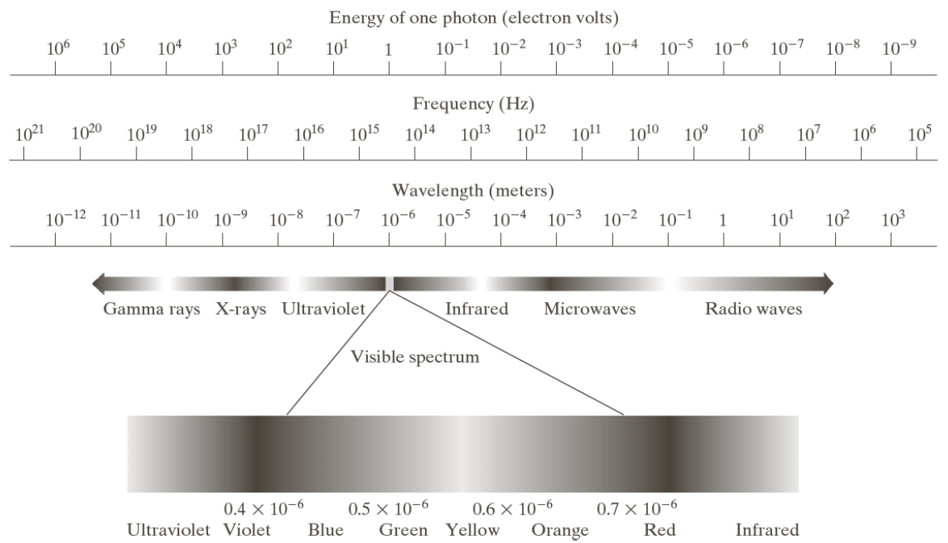# Digital image fundamentals

Institute for Environmental and Spatial Analysis...University of North Georgia

## 1   Principal energy source for digital images

Principal energy source for images: Electromagnetic (EM) energy spectrum.

EM waves: Stream of massless particles (protons), each traveling in a wave-like pattern at the speed of light

Each massless particle has a bundle of energy called photonFigures from Gonzalez and Woods (2002)

## 2   Waves

Wavelength: $\lambda=\frac{c}{\nu}$

• $c$: Speed of light ($2.998\times 10^8 \mathrm{m/s}$)
• $\nu$: Frequency in Hz
• 1 Hz is 1 cycle of a sinusoidal wave per second
• Units of wavelength: m, microns ($\mathrm{\mu m}, 10^{-6} \mathrm{m}$), nanometers (nm, $10^{-9} \mathrm{m}$)

Energy: $E=h\nu$

• $h$: Planck’s constant ($4.135,667,66\times 10^{-15} \mathrm{eV}$)
• Units of energy: electron-volt (eV)

## 3   Alternative energy sources

Acoustic

• Geological applications: Mineral and oil exploration using low-frequency waves (e.g., 100 Hz)
• Low-frequency waves travel a longer distance because they lose less energy to the medium (less active $\rightarrow$ less loss)

Ultrasound

• Medical applications: Baby
• High-frequency waves (highly active $\rightarrow$ high loss)

Electronic

• Electron microscopy

## 4   Imaging sensors

• Single imaging sensor (point)
• Line sensor (linear)
• Array sensor (rectangular)

## 5   Digital image

$f(x,y)=i(x,y)\times r(x,y)$

• $i(x,y) \in [0,\infty)$: Illumination, characteristics of the energy source
• $r(x,y) \in [0,1]$: Reflectance, characteristics of the object sensed

## 6   Image sampling and quantizationFigures from Gonzalez and Woods (2002)

## 7   Representation of digital images

$M$ rows, $N$ columns

A digital image $=$ An $M$-by-$N$ matrix

By Python convention (because we use Python)

• Rows: $0,1,\cdots,M-1$
• Columns: $0,1,\cdots,N-1$

$f(x,y)=\begin{bmatrix} f(0,0)& \cdots& f(0,N-1)\\ \vdots& \ddots& \vdots\\ f(M-1,0)& \cdots& f(M-1,N-1) \end{bmatrix}$

$\mathbf{A}=\begin{bmatrix} a_{0,0}& \cdots& a_{0,N-1}\\ \vdots& \ddots& \vdots\\ a_{M-1,0}& \cdots& a_{M-1,N-1} \end{bmatrix}$

## 8   BitsLSB: Least significant bit

MSB: Most significant bit

1 byte $=$ 8 bits

Unsigned integer $\in [2^0,2^8-1]=[0,255]$

What is this number in decimal notation?

## 9   Gray levels

Number of bits per pixel: $k$

Gray levels: $L=2^k$

$f(x,y)=\begin{cases} 0& \text{black}\\ L-1& \text{white} \end{cases}$

$k$$L$Description
12Binary image in black and white
66464 gray levels, limit of human visual system
8256Typical gray level resolution (1 byte per pixel)

## 10   Storage bits and bytes

Storage bits: $b=M\times N\times k$

Storage bytes: $B=\left\lceil\frac{b}{8}\right\rceil$

Problems

1. $M=N=32, L=2$
2. $M=N=32, L=128$

## 11   Spatial and gray-level resolution

$M$ rows, $N$ columns, $L$ gray levels

Spatial resolution: $M\times N$ pixels, sometimes in pixels per inch (ppi)

Gray resolution: $L$ levels

Convention: $M\times N, L$-level image

For example,

• $512\times 512, 8$-level image
• $1024\times 1024, 256$-level image

## 12   Homework: Wavelength and image storage

1. The frequency of commercial alternating current in the United States is 60 Hz. Find its wavelength.
2. How many storage bits and bytes do you need to store a digital image of size 128-by-128 pixels and 256 gray levels?

Show your work for full credits!